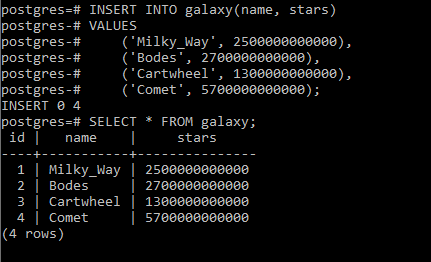GeeksforGeeks App
Open AppBrowser
Continue

# PostgreSQL – BIGINT Integer Data Type

PostgreSQL allows a type of integer type namely `BIGINT`. It requires 8 bytes of storage size and can store integers in the range of -9, 223, 372, 036, 854, 775, 808 to +9, 223, 372, 036, 854, 775, 807. Using BIGINT type is not only consuming a lot of storage but also decreasing the performance of the database, therefore, you should have a good reason to use it. It comes in handy for storing data like the number of stars in a galaxy, the scientific constants, etc.

`Syntax: variable_name BIGINT`

Now let’s look into some examples of use cases of SMALLINT integer type.
Example 1:
In this example we will create a table that stores the number of stars in various galaxies by using the below commands:

```CREATE TABLE galaxy(
id SERIAL PRIMARY KEY,
name VARCHAR (255) NOT NULL,
stars BIGINT NOT NULL CHECK (stars> 0)
);```

Now let’s add some data to the table using the below command:

```INSERT INTO galaxy(name, stars)
VALUES
('Milky_Way', 2500000000000),
('Bodes', 2700000000000),
('Cartwheel', 1300000000000),
('Comet', 5700000000000);```

Now let’s check our inserted data using the below commands:

`SELECT * FROM galaxy;`

Output:Example 2:
In this example we will create a table that stores the value of various scientific constants by using the below commands:

```CREATE TABLE constants(
id SERIAL PRIMARY KEY,
name VARCHAR (255) NOT NULL,
value BIGINT NOT NULL CHECK (value> 0)
);```

Now let’s add some data to the table using the below command:

```INSERT INTO constants(name, value)
VALUES
('Mole', 602213950000000000),
('Rydberg_constant', 10973731568525000),
`SELECT * FROM constants;`